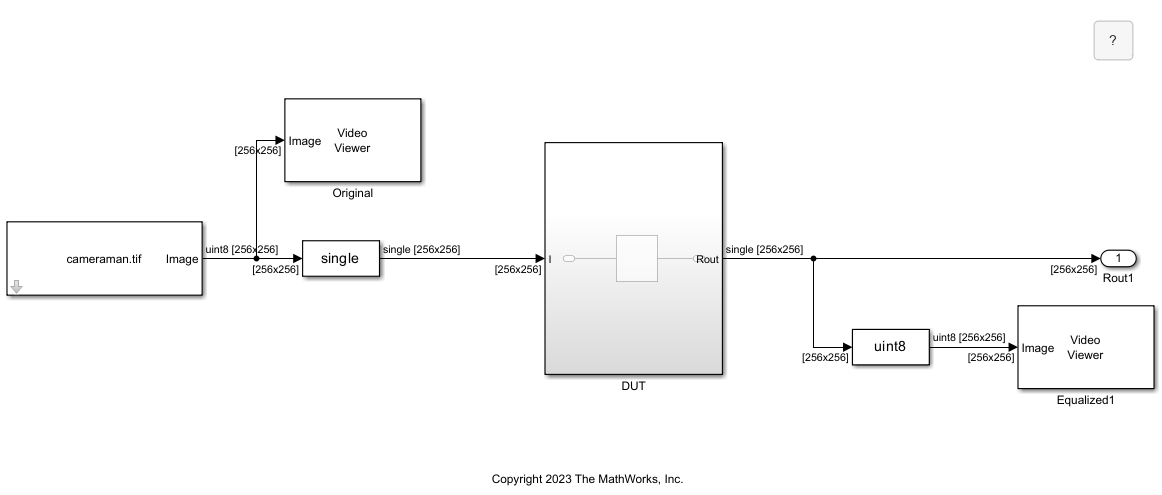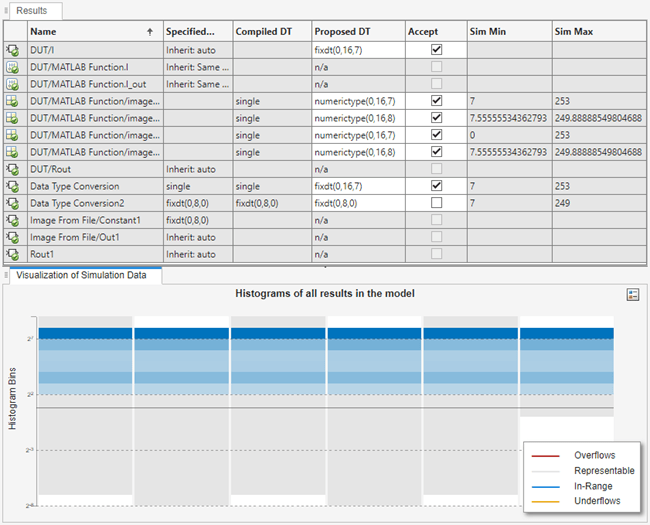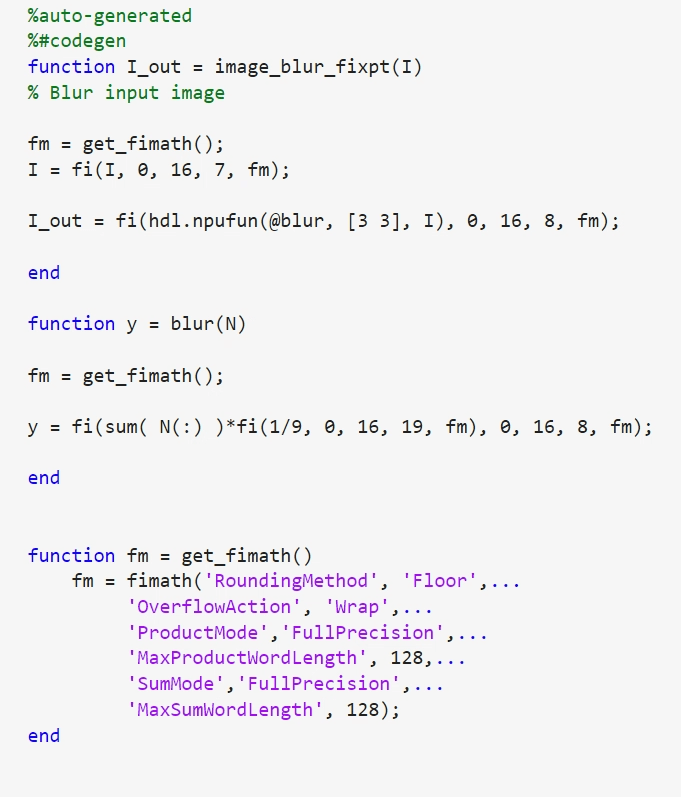# Convert Frame-Based Model to Fixed Point and Generate HDL Code

This example shows how to use the Fixed-Point Tool to convert the data types in a frame-based model to fixed point and generate HDL code using frame-to-sample conversion. Combining fixed-point conversion with frame-to-sample conversion allows you to generate HDL code from frame-based models using fixed-point arithmetic, which can be more efficient and suitable for hardware implementations. To learn more about generating HDL code with frame-to-sample conversion, see Generate HDL Code from a Frame-Based Model Example (HDL Coder).

The model `hdlFrame_Blur_2D_MLFB` uses a common image-processing frame-based blurring algorithm that uses a neighborhood processing pattern. The model applies an image-blurring kernel to the image `cameraman.tif`. Open the model to view the frame-based blurring algorithm.

`open_system("hdlFrame_Blur_2D_MLFB")`

To view the data types of the signals before fixed-point conversion, on the Debug tab, select Information Overlays > Base Data Types.The `DUT` subsystem contains the MATLAB® function `image_blur` in the MATLAB Function block. The `image_blur` function calls the frame-to-sample supported function `hdl.npufun` (HDL Coder), which applies the blurring kernel in the `blur` function to the input image.

`open_system("hdlFrame_Blur_2D_MLFB/DUT/MATLAB Function")`### Convert to Fixed Point

To convert the model to fixed point, on the Apps tab, in the Code Generation section, select the Fixed-Point Tool.

When Fixed-Point Tool launches, select Iterative Fixed Point Conversion.

Select the model `hdlFrame_Blur_2D_MLFB` as the system under design. For Range Collection Mode, select Simulation ranges.In the Iterative Fixed-Point Conversion toolstrip, click Collect Ranges. After collecting ranges, click Propose Data Types.Select the check box for each of the proposed data types you would like to accept, then click Apply Data Types.

The attached model `hdlFrame_Blur_2D_MLFB_fixpt` contains the resulting fixed-point model. Once you apply the fixed-point data types, your model should match the attached model. Open the attached fixed-point model.

`open_system("hdlFrame_Blur_2D_MLFB_fixpt");`

Open the MATLAB Function block to view the changes after fixed-point conversion.

`open_system("hdlFrame_Blur_2D_MLFB_fixpt/DUT/MATLAB Function")`

A variant subsystem is automatically generated by the Fixed-Point Tool to convert the MATLAB Function block to fixed point. The variant subsystem contains two blocks:

1. `MATLAB Function` is the original code from the MATLAB Function block.

2. `MATLAB Function_FixPt` is the generated fixed-point code for the MATLAB Function block

The `image_blur` function has been converted to fixed point and saved as a new function, `image_blur_fixpt`, within the `MATLAB Function_FixPt` block.View the new `image_blur_fixpt` function by opening the `MATLAB Function_FixPt` block.

`open_system("hdlFrame_Blur_2D_MLFB_fixpt/DUT/MATLAB Function/MATLAB Function_FixPt")`To inspect the data types in `image_blur_fixpt`, on the Function tab, click Function Review. Pause on a line of the function to view details about the fixed-point type. For more information about reviewing functions, see MATLAB Function Reports.### Generate HDL Code Using Frame-to-Sample Conversion

Generate HDL code following the steps in Generate HDL Code from a Frame-Based Model Example (HDL Coder).

Apply the frame-to-sample conversion optimization by setting the model configuration parameter `FrameToSampleConversion` to `on`.

`hdlset_param("hdlFrame_Blur_2D_MLFB_fixpt", FrameToSampleConversion = "on");`

Specify which incoming frame-based signal to convert to a sample-based signal by setting the HDL Inport block property `ConvertToSamples` of the input to `on`. In this example, set the `ConvertToSamples` property to `on` for the only Inport block to the `DUT` subsystem, `I`.

`hdlset_param("hdlFrame_Blur_2D_MLFB_fixpt/DUT/I", ConvertToSamples = "on");`

Generate HDL code from the `DUT` subsystem. Compare the output of HDL code generation from the fixed-point model to the output of the floating-point model in Generate HDL Code from a Frame-Based Model Example. Applying fixed-point conversion to the model reduces the output latency of HDL code generation from 127 cycles to 7 cycles.

```hdlset_param("hdlFrame_Blur_2D_MLFB_fixpt", GenerateModel = "on"); makehdl('hdlFrame_Blur_2D_MLFB_fixpt/DUT');```
```### Begin compilation of the model 'hdlFrame_Blur_2D_MLFB_fixpt'... ### Working on the model hdlFrame_Blur_2D_MLFB_fixpt ### Generating HDL for hdlFrame_Blur_2D_MLFB_fixpt/DUT ### Using the config set for model hdlFrame_Blur_2D_MLFB_fixpt for HDL code generation parameters. ### Running HDL checks on the model 'hdlFrame_Blur_2D_MLFB_fixpt'. ### Working on the model 'hdlFrame_Blur_2D_MLFB_fixpt'... ### The code generation and optimization options you have chosen have introduced additional pipeline delays. ### The delay balancing feature has automatically inserted matching delays for compensation. ### The DUT requires an initial pipeline setup latency. Each output port experiences these additional delays. ### Output port 1: 7 cycles. ### Output port 1: The first valid output of this port will be after an initial latency of 257 valid inputs. ### Output port 2: 7 cycles. ### Output port 2: The first valid output of this port will be after an initial latency of 257 valid inputs. ### Working on... GenerateModel ### Begin model generation 'gm_hdlFrame_Blur_2D_MLFB_fixpt'... ### Rendering DUT with optimization related changes (IO, Area, Pipelining)... ### Model generation complete. ### Generated model saved at hdlsrc\hdlFrame_Blur_2D_MLFB_fixpt\gm_hdlFrame_Blur_2D_MLFB_fixpt.slx ### Begin VHDL Code Generation for 'hdlFrame_Blur_2D_MLFB_fixpt'. ### MESSAGE: The design requires 65536 times faster clock with respect to the base rate = 1. ### Working on counterNetwork as hdlsrc\hdlFrame_Blur_2D_MLFB_fixpt\counterNetwork.vhd. ### Working on NeighborhoodCreator_3x3/row3_col2 as hdlsrc\hdlFrame_Blur_2D_MLFB_fixpt\row3_col2.vhd. ### Working on NeighborhoodCreator_3x3/row2_linebuffer/SimpleDualPortRAM_generic as hdlsrc\hdlFrame_Blur_2D_MLFB_fixpt\SimpleDualPortRAM_generic.vhd. ### Working on NeighborhoodCreator_3x3/row2_linebuffer as hdlsrc\hdlFrame_Blur_2D_MLFB_fixpt\row2_linebuffer.vhd. ### Working on NeighborhoodCreator_3x3 as hdlsrc\hdlFrame_Blur_2D_MLFB_fixpt\NeighborhoodCreator_3x3.vhd. ### Working on boundaryCounters_3_3 as hdlsrc\hdlFrame_Blur_2D_MLFB_fixpt\boundaryCounters_3_3.vhd. ### Working on BoundaryCheck_3x3 as hdlsrc\hdlFrame_Blur_2D_MLFB_fixpt\BoundaryCheck_3x3.vhd. ### Working on I_NeighborhoodCreator as hdlsrc\hdlFrame_Blur_2D_MLFB_fixpt\I_NeighborhoodCreator.vhd. ### Working on hdlFrame_Blur_2D_MLFB_fixpt/DUT/MATLAB Function/MATLAB Function_FixPt/blur as hdlsrc\hdlFrame_Blur_2D_MLFB_fixpt\blur.vhd. ### Working on hdlFrame_Blur_2D_MLFB_fixpt/DUT/MATLAB Function/MATLAB Function_FixPt as hdlsrc\hdlFrame_Blur_2D_MLFB_fixpt\MATLAB_Function_FixPt.vhd. ### Working on hdlFrame_Blur_2D_MLFB_fixpt/DUT/MATLAB Function as hdlsrc\hdlFrame_Blur_2D_MLFB_fixpt\MATLAB_Function.vhd. ### Working on hdlFrame_Blur_2D_MLFB_fixpt/DUT/Input_FIFOs/I_FIFO as hdlsrc\hdlFrame_Blur_2D_MLFB_fixpt\I_FIFO.vhd. ### Working on hdlFrame_Blur_2D_MLFB_fixpt/DUT/Input_FIFOs as hdlsrc\hdlFrame_Blur_2D_MLFB_fixpt\Input_FIFOs.vhd. ### Working on hdlFrame_Blur_2D_MLFB_fixpt/DUT/Output_FIFOs/MATLAB Function_out1_FIFO as hdlsrc\hdlFrame_Blur_2D_MLFB_fixpt\MATLAB_Function_out1_FIFO.vhd. ### Working on hdlFrame_Blur_2D_MLFB_fixpt/DUT/Output_FIFOs as hdlsrc\hdlFrame_Blur_2D_MLFB_fixpt\Output_FIFOs.vhd. ### Working on hdlFrame_Blur_2D_MLFB_fixpt/DUT as hdlsrc\hdlFrame_Blur_2D_MLFB_fixpt\DUT.vhd. ### Generating package file hdlsrc\hdlFrame_Blur_2D_MLFB_fixpt\DUT_pkg.vhd. ### Code Generation for 'hdlFrame_Blur_2D_MLFB_fixpt' completed. ### Generating HTML files for code generation report at hdlFrame_Blur_2D_MLFB_fixpt_codegen_rpt.html ### Creating HDL Code Generation Check Report DUT_report.html ### HDL check for 'hdlFrame_Blur_2D_MLFB_fixpt' complete with 0 errors, 4 warnings, and 1 messages. ### HDL code generation complete. ```